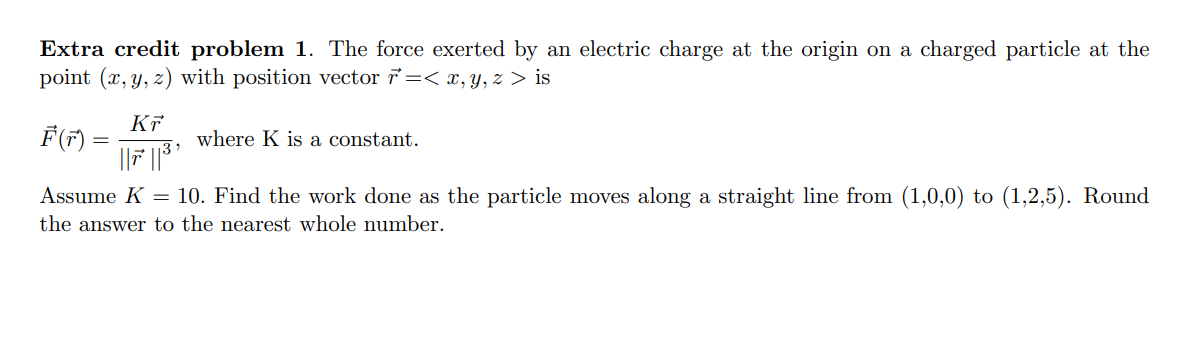### Create an Account

Already have account?

### Forgot Your Password ?

Home / Questions / Extra credit problem 1. The force exerted by an electric charge at the origin on a charged...

# Extra credit problem 1. The force exerted by an electric charge at the origin on a charged particle at the point (x, y, z) with position vector r = is Kr F(7) where K is a constant. 117 118? Assume K

Extra credit problem 1. The force exerted by an electric charge at the origin on a charged particle at the point (x, y, z) with position vector r = is Kr F(7) where K is a constant. 117 118? Assume K = 10. Find the work done as the particle moves along a straight line from (1,0,0) to (1,2,5). Round the answer to the nearest whole numberApr 13 2021 View more View Less

#### Answer (Solved)Subscribe To Get Solution